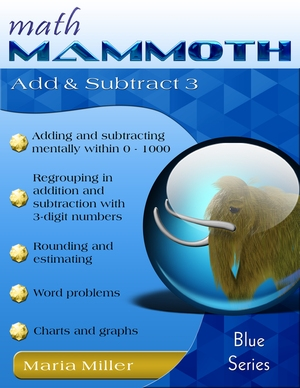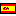^
You are here: HomeBlue Series → Add & Subtract 3

# Math Mammoth Add & Subtract 3115 pages

## Prices & ordering

Printed copy \$15.05

Printed copy £7.20, full color
US shipping availableEste libro en español

Math Mammoth Add and Subtract 3 has to do with adding and subtracting 3-digit numbers. The book is suitable to study after the student has learned to add and subtract with 2-digit numbers, and has learned numbers till 1,000. The goal is to teach the student to add and subtract both mentally and in columns within 0-1000.

The PDF version of this book is enabled for annotation. This means that if you prefer, your student can fill it in on the computer, using the typewriter and drawing tools in Adobe Reader version 9 or greater.

Since students usually learn three-digit numbers in second grade, some of the topics in this book suit second grade, some suit third grade.

The first section of the book explores some mental math with three-digit numbers, and is suitable for second grade. We study adding and subtracting whole hundreds, whole tens, and ones within 0-1000, often comparing to similar problems within 0-100. In most of these lessons, the addition or subtraction is first illustrated with a visual model. You can use manipulatives instead, if you prefer.

The next part (the middle part) deals with adding and subtracting in columns with regrouping. If you want to follow the grade levels, these lessons are intended for both second and third grade this way: the lessons are for second grade, until the topics of regrouping twice in subtraction and regrouping over zero tens in subtraction, which are for third grade.

The processes of regrouping in addition and subtraction are first practiced using visual models, to ensure the student understands the concept (the "why"), and does not only learn the procedure (the "how").

Regrouping in subtraction with three-digit numbers includes three different cases:

1. Regrouping 1 ten as 10 ones, such as is needful for 546 − 229.
2. Regrouping 1 hundred as 10 tens, such as is needful for 728 − 441.
3. Regrouping two times (1 ten as 10 ones, and 1 hundred as 10 tens), such as is needful for 725 − 448.
4. Regrouping with zero tens, such as is needful for 405 − 278. Here, first we regroup 1 hundred as 10 tens, then 1 ten as 10 ones.

In the last section, the book includes addition and subtraction topics for third grade, starting with mental addition and subtraction. Through it all, students solve lots of word problems and practice some algebra in disguise, where they use a symbol or a ? for the unknown thing in the problem. We also study ordinal numbers, rounding and estimating, and order of operations.

Then we study the connection between addition and subtraction with bigger numbers, which also aims to help children think algebraically. Lastly, students get to practice their adding and subtracting skills in a practical way through reading a mileage chart and other types of graphs.

The PDF version of this book is enabled for annotation. This means that if you prefer, your student can fill it in on the computer, using the typewriter and drawing tools in Adobe Reader version 9 or greater.

You might also be interested in:
• Math Mammoth Add & Subtract 4—a worktext about mental addition and subtraction strategies, regrouping, Roman numerals, the connection between addition and subtraction, order of operations, bar graphs, line graphs, and money problems (grades 3-4).
• Math Mammoth Place Value 2—a worktext about three-digit numbers (place value), comparing, ordering, rounding, bar graphs, and pictographs (grade 2).
• Math Mammoth Place Value 3—a worktext about four-digit place value, adding & subtracting 4-digit numbers mentally, rounding, estimating, and order of operations (grade 3).
• Make It Real Learning: Arithmetic I—an activity workbook with real-world data, focusing on situations that use addition, subtraction, estimation, division, bar graphs, and place value with large numbers (grades 3-6).

## Better Yet - Bundle Deals!

*BONUS*: Buy any bundle listed above, and get the fully upgraded Soft-Pak software for FREE (includes 4 math and 2 language arts programs, complete with on screen and printable options).

## How and where to order

You can buy Math Mammoth books at:

• Here at MathMammoth.com website — simply use the "Add to cart" buttons you see on the product pages.
• Rainbow Resource carries printed copies for the Light Blue series books, plus several CDs (Light Blue and Blue series).
• Homeschool Buyers Co-op offers download versions of the Light Blue series, plus the Blue series bundle.
• At Teachers Pay Teachers you can purchase the Light Blue Series downloads, plus topical units.
• Lulu sells printed copies for most of the Math Mammoth materials (various series).

By purchasing any of the books, permission IS granted for the teacher (or parent) to reproduce this material to be used with his/her students in a teaching situation; not for commercial resale. However, you are not permitted to share the material with another teacher.

In other words, you are permitted to make copies for the students/children you are teaching, but not for other teachers' usage.

Math Mammoth books are PDF files. You will need Adobe Reader to view them, including if you use a Mac or Linux. You can try other PDF viewers, but they seem to either omit or mess up some of the images.
WAIT!

Receive my monthly collection of math tips & resources directly in your inbox — and get a FREE Math Mammoth book!You can unsubscribe at any time.

### Math Mammoth Tour

Confused about the different options? Take a virtual email tour around Math Mammoth! You'll receive:

An initial email to download your GIFT of over 400 free worksheets and sample pages from my books. Six other "TOURSTOP" emails that explain the important things and commonly asked questions concerning Math Mammoth curriculum. (Find out the differences between all these different-colored series!)

This way, you'll have time to digest the information over one or two weeks, plus an opportunity to ask me personally about the curriculum.
A monthly collection of math teaching tips & Math Mammoth updates (unsubscribe any time)### "Mini" Math Teaching Course

This is a little "virtual" 2-week course, where you will receive emails on important topics on teaching math, including:

- How to help a student who is behind
- Troubles with word problems
- Teaching multiplication tables
- Why fractions are so difficult
- The value of mistakes
- Should you use timed tests
- And more!

A GIFT of over 400 free worksheets and sample pages from my books right in the very beginning.A monthly collection of math teaching tips & Math Mammoth updates (unsubscribe any time)
Enter your email to receive math teaching tips, resources, Math Mammoth news & sales, humor, and more! I tend to send out these tips about once monthly, near the beginning of the month, but occasionally you may hear from me twice per month (and sometimes less often).• A GIFT of over 400 free worksheets and sample pages from my books.